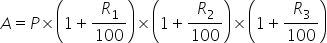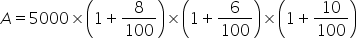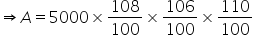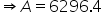Maths-
General
Easy

Question

# Find the compound interest for 3 years on Rs 5000, if the rate of interest for the successive years are 8%, 6% and 10% respectively.Hint:

## The correct answer is: 1296.4 Rupees

### Complete step by step solution:Given that principal amount P = 5000Number of years T = 3Let R1 = 8%,R2 = 6% and R3 = 10%Total amount ,…(i)On substituting the known values in (i), we getWe know that, Compound interest ( CI) = total amount (A) - principal amount (P)So, Compound interest ( CI) = 6296.4 - 5000 = 1296.4 Rupees#### With Turito Foundation.#### Get an Expert Advice From Turito.